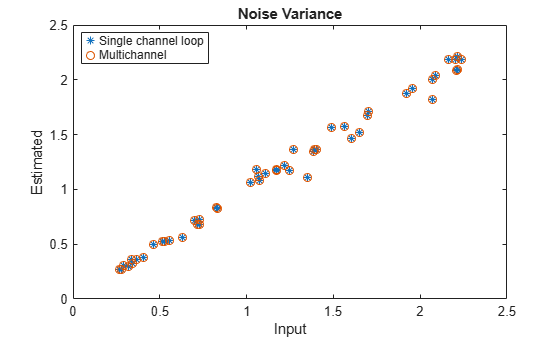Documentation

# arburg

Autoregressive all-pole model parameters — Burg’s method

## Syntax

```a = arburg(x,p) [a,e] = arburg(x,p) [a,e,rc] = arburg(x,p) ```

## Description

`a = arburg(x,p)` returns the normalized autoregressive (AR) parameters corresponding to a model of order `p` for the input array, `x`. If `x` is a vector, then the output array, `a`, is a row vector. If `x` is a matrix, then the parameters along the nth row of `a` model the nth column of `x`. `a` has `p` + 1 columns. `p` must be less than the number of elements (or rows) of `x`.

`[a,e] = arburg(x,p)` returns the estimated variance, `e`, of the white noise input.

`[a,e,rc] = arburg(x,p)` returns the reflection coefficients in `rc`.

## Examples

collapse all

Use a vector of polynomial coefficients to generate an AR(4) process by filtering 1024 samples of white noise. Reset the random number generator for reproducible results. Use Burg's method to estimate the coefficients.

```rng default A = [1 -2.7607 3.8106 -2.6535 0.9238]; y = filter(1,A,0.2*randn(1024,1)); arcoeffs = arburg(y,4)```
```arcoeffs = 1×5 1.0000 -2.7743 3.8408 -2.6843 0.9360 ```

Generate 50 realizations of the process, changing each time the variance of the input noise. Compare the Burg-estimated variances to the actual values.

```nrealiz = 50; noisestdz = rand(1,nrealiz)+0.5; randnoise = randn(1024,nrealiz); for k = 1:nrealiz y = filter(1,A,noisestdz(k) * randnoise(:,k)); [arcoeffs,noisevar(k)] = arburg(y,4); end plot(noisestdz.^2,noisevar,'*') title('Noise Variance') xlabel('Input') ylabel('Estimated')```Repeat the procedure using `arburg`'s multichannel syntax.

```realiz = bsxfun(@times,noisestdz,randnoise); Y = filter(1,A,realiz); [coeffs,variances] = arburg(Y,4); hold on plot(noisestdz.^2,variances,'o') q = legend('Single channel loop','Multichannel'); q.Location = 'best';```collapse all

### AR(p) Model

In an AR model of order p, the current output is a linear combination of the past p outputs plus a white noise input. The weights on the p past outputs minimize the mean-square prediction error of the autoregression. If y(n) is the current value of the output and x(n) is a zero mean white noise input, the AR(p) model is:

`$y\left(n\right)+\sum _{k=1}^{p}a\left(k\right)y\left(n-k\right)=x\left(n\right).$`

### Reflection Coefficients

The reflection coefficients are the partial autocorrelation coefficients scaled by –1. The reflection coefficients indicate the time dependence between y(n) and y(n – k) after subtracting the prediction based on the intervening k – 1 time steps.

## Algorithms

The Burg method estimates the reflection coefficients and uses the reflection coefficients to estimate the AR parameters recursively. You can find the recursion and lattice filter relations describing the update of the forward and backward prediction errors in .

## References

 Kay, Steven M. Modern Spectral Estimation: Theory and Application. Englewood Cliffs, NJ: Prentice Hall, 1988.